Home > LSCF, Statistics > Lines, Sines, and Curve Fitting 16: Wavelets

Lines, Sines, and Curve Fitting 16: Wavelets

2011 February 20

Wherein I extend the simple Fourier analysis in Lines, Sines, and Curve Fitting 15 – Periodic Noise to Morlet Wavelet analysis via the R package dplR, also known as the “Dendrochronology Program Library in R”

Take a sign wave with a monotonically increasing period. Period at the beginning of the series is 45 years. Every period thereafter increases by 5 years: 45,50,55,60,65,70,and a bit of 75.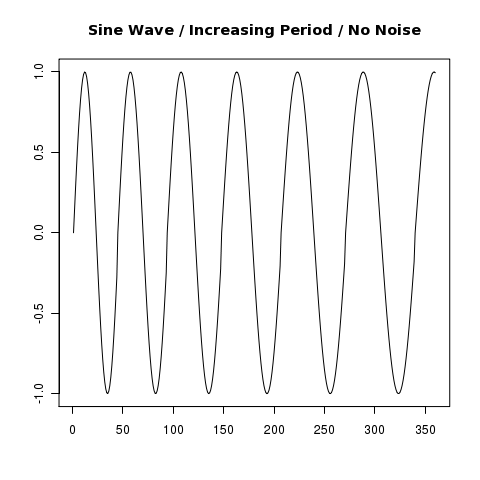Our old friend, the Fourier transform, appears to give a pretty sharp spike for the period … but what is the period?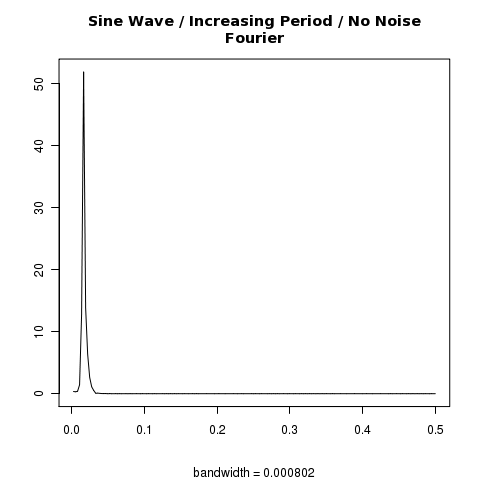Looking at various intensity thresholds, we see different results:
> 1/sp\$freq[sp\$spec>2]
 72.00000 60.00000 51.42857 45.00000 40.00000
> 1/sp\$freq[sp\$spec>10]
 72.00000 60.00000 51.42857
> 1/sp\$freq[sp\$spec>20]
 60
> 1/sp\$freq[sp\$spec>30]
 60

Sixty years might not be wrong, but it isn’t right either. We know that the period varies from 45 to 75 years; we coded it that way. So while 60 might be a reasonable average, it doesn’t really give us the flavor of the period changes over time. For that, we turn to the Morlet wavelet analysis.

Below we graph the Morlet Wavelet analysis. Notice that the period intensity is shown as a band of values (see red bands) that vary (slowly increasing) over time.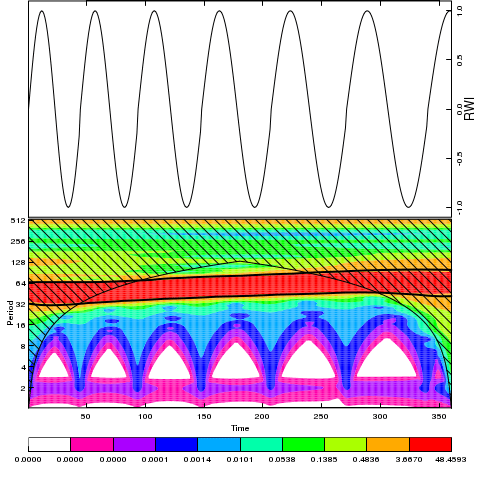The package also allows us to display the probability related with the various periods tested. In the following chart: white = 0% – 50%, blue = 50% – 75%, green = 75% – 90%, and red = 90% – 99%.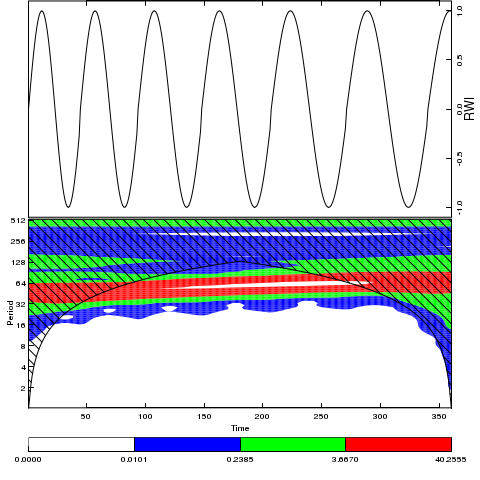We repeat the above with a noisy amplitude and gaussian noise added to each sample point.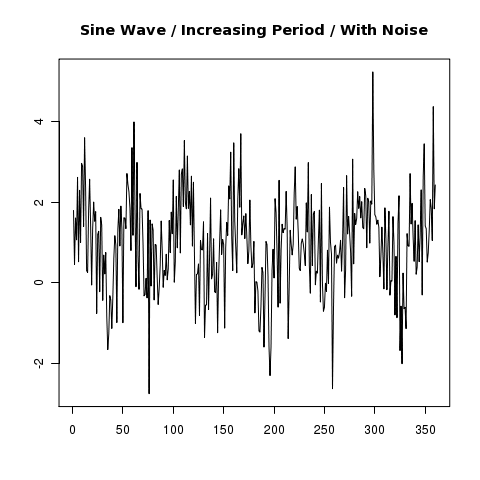The fourier spectrum intensity.Note that the added noise generates spurious low frequency hits* and broadens the power spectrum band, leading to more hits at a given intensity threshold.
> 1/sp\$freq[sp\$spec>2]
 180.000000 72.000000 60.000000 51.428571 45.000000 36.000000
 30.000000 18.000000 11.250000 10.285714 9.473684 9.000000
 8.780488 5.714286 5.625000 5.217391 4.615385 4.556962
 3.272727 3.157895 2.706767 2.608696 2.571429 2.278481
 2.022472
> 1/sp\$freq[sp\$spec>10]
 60.00000 51.42857 45.00000
> 1/sp\$freq[sp\$spec>20]
 60
> 1/sp\$freq[sp\$spec>30]
 60
> #  60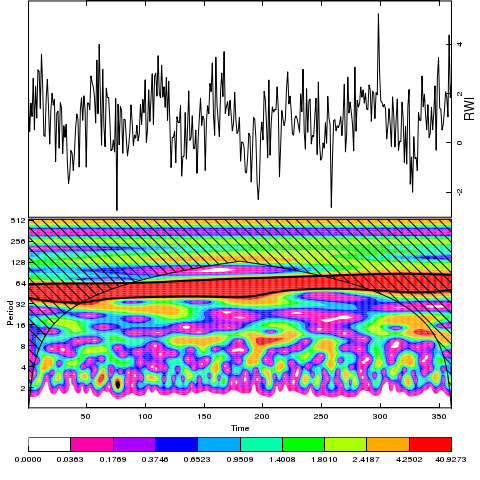* Notice that the spurious low frequency noise generates fairly high probabilities (> 50%). Recall: white = 0% – 50%, blue = 50% – 75%, green = 75% – 90%, and red = 90% – 99%.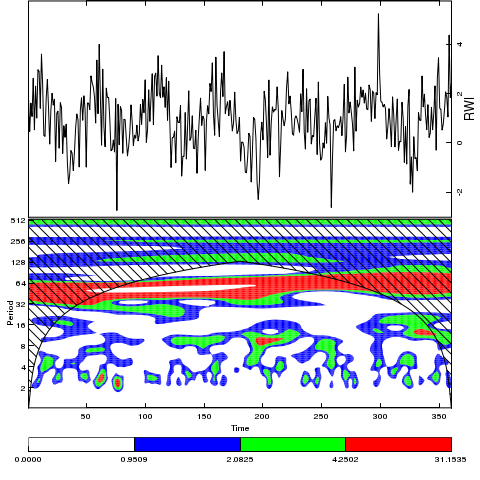Script is here

Update: Noticed this comment from Aug 2010 regarding spurious low freq noise. Had to tweak the code slightly.

library(“dplR”)
m1 <- morlet(y=rnorm(99,0,82),x=1901:1999,p2=5,siglvl=0.95)
wavelet.plot(m1)

m2 <- morlet(arima.sim(list(order = c(1,0,0),ar=.1), n = 99, rand.gen=rnorm, sd=82),1901:1999,p2=5,siglvl=0.95)
wavelet.plot(m2)